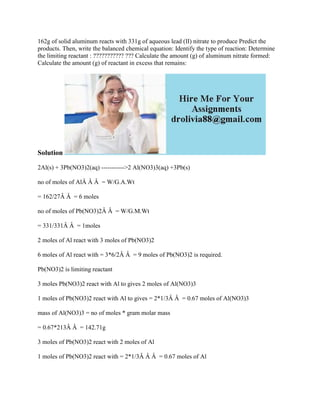Diese Präsentation wurde erfolgreich gemeldet.

# 162g of solid aluminum reacts with 331g of aqueous lead (II) nitrate t.docx

Anzeige
Anzeige
Anzeige
Anzeige
Anzeige
Anzeige
Anzeige
Anzeige
Anzeige
Anzeige
Anzeige×

1 von 2 Anzeige

# 162g of solid aluminum reacts with 331g of aqueous lead (II) nitrate t.docx

162g of solid aluminum reacts with 331g of aqueous lead (II) nitrate to produce Predict the products. Then, write the balanced chemical equation: Identify the type of reaction: Determine the limiting reactant : ??????????? ??? Calculate the amount (g) of aluminum nitrate formed: Calculate the amount (g) of reactant in excess that remains:
Solution
2Al(s) + 3Pb(NO3)2(aq) ----------->2 Al(NO3)3(aq) +3Pb(s)
no of moles of AlÂ Â Â  = W/G.A.Wt
= 162/27Â Â  = 6 moles
no of moles of Pb(NO3)2Â Â  = W/G.M.Wt
= 331/331Â Â  = 1moles
2 moles of Al react with 3 moles of Pb(NO3)2
6 moles of Al react with = 3*6/2Â Â  = 9 moles of Pb(NO3)2 is required.
Pb(NO3)2 is limiting reactant
3 moles Pb(NO3)2 react with Al to gives 2 moles of Al(NO3)3
1 moles of Pb(NO3)2 react with Al to gives = 2*1/3Â Â  = 0.67 moles of Al(NO3)3
mass of Al(NO3)3 = no of moles * gram molar mass
= 0.67*213Â Â  = 142.71g
3 moles of Pb(NO3)2 react with 2 moles of Al
1 moles of Pb(NO3)2 react with = 2*1/3Â Â Â  = 0.67 moles of Al
The no of moles of excess reactant remains after complete the reaction = 6-0.67Â Â  = 5.33 moles of Al
The amount of excess reactant remains after complete the reaction = 5.33*27Â Â  = 143.91g of Al
.

162g of solid aluminum reacts with 331g of aqueous lead (II) nitrate to produce Predict the products. Then, write the balanced chemical equation: Identify the type of reaction: Determine the limiting reactant : ??????????? ??? Calculate the amount (g) of aluminum nitrate formed: Calculate the amount (g) of reactant in excess that remains:
Solution
2Al(s) + 3Pb(NO3)2(aq) ----------->2 Al(NO3)3(aq) +3Pb(s)
no of moles of AlÂ Â Â  = W/G.A.Wt
= 162/27Â Â  = 6 moles
no of moles of Pb(NO3)2Â Â  = W/G.M.Wt
= 331/331Â Â  = 1moles
2 moles of Al react with 3 moles of Pb(NO3)2
6 moles of Al react with = 3*6/2Â Â  = 9 moles of Pb(NO3)2 is required.
Pb(NO3)2 is limiting reactant
3 moles Pb(NO3)2 react with Al to gives 2 moles of Al(NO3)3
1 moles of Pb(NO3)2 react with Al to gives = 2*1/3Â Â  = 0.67 moles of Al(NO3)3
mass of Al(NO3)3 = no of moles * gram molar mass
= 0.67*213Â Â  = 142.71g
3 moles of Pb(NO3)2 react with 2 moles of Al
1 moles of Pb(NO3)2 react with = 2*1/3Â Â Â  = 0.67 moles of Al
The no of moles of excess reactant remains after complete the reaction = 6-0.67Â Â  = 5.33 moles of Al
The amount of excess reactant remains after complete the reaction = 5.33*27Â Â  = 143.91g of Al
.

Anzeige
Anzeige

### 162g of solid aluminum reacts with 331g of aqueous lead (II) nitrate t.docx

1. 1. 162g of solid aluminum reacts with 331g of aqueous lead (II) nitrate to produce Predict the products. Then, write the balanced chemical equation: Identify the type of reaction: Determine the limiting reactant : ??????????? ??? Calculate the amount (g) of aluminum nitrate formed: Calculate the amount (g) of reactant in excess that remains: Solution 2Al(s) + 3Pb(NO3)2(aq) ----------->2 Al(NO3)3(aq) +3Pb(s) no of moles of AlÂ Â Â = W/G.A.Wt = 162/27Â Â = 6 moles no of moles of Pb(NO3)2Â Â = W/G.M.Wt = 331/331Â Â = 1moles 2 moles of Al react with 3 moles of Pb(NO3)2 6 moles of Al react with = 3*6/2Â Â = 9 moles of Pb(NO3)2 is required. Pb(NO3)2 is limiting reactant 3 moles Pb(NO3)2 react with Al to gives 2 moles of Al(NO3)3 1 moles of Pb(NO3)2 react with Al to gives = 2*1/3Â Â = 0.67 moles of Al(NO3)3 mass of Al(NO3)3 = no of moles * gram molar mass = 0.67*213Â Â = 142.71g 3 moles of Pb(NO3)2 react with 2 moles of Al 1 moles of Pb(NO3)2 react with = 2*1/3Â Â Â = 0.67 moles of Al
2. 2. The no of moles of excess reactant remains after complete the reaction = 6-0.67Â Â = 5.33 moles of Al The amount of excess reactant remains after complete the reaction = 5.33*27Â Â = 143.91g of Al